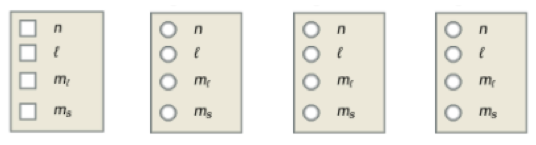# Problem: Answer each of the following questions.a) What information is needed to determine the energy of an electron in a many-electron atom? Check all that apply.b) What information is most important in determining the size of an orbital? c) What information is needed to determine the orientation of an orbital? d) What information is needed to determine the general shape of an orbital?

🤓 Based on our data, we think this question is relevant for Professor Young's class at NOTRE DAME.

###### FREE Expert Solution

a) Principle quantum number $n$, which determines the size and energy, is needed to determine the energy of an electron in a many-electron atom.

b) Principle quantum number $n$ is needed to determine the size of an orbital.###### Problem Details

Answer each of the following questions.

a) What information is needed to determine the energy of an electron in a many-electron atom? Check all that apply.

b) What information is most important in determining the size of an orbital?

c) What information is needed to determine the orientation of an orbital?

d) What information is needed to determine the general shape of an orbital?